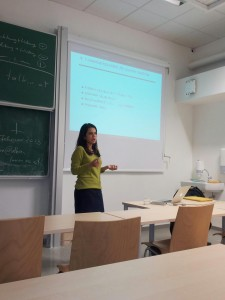# Samanta Roopsha

## Robustness Analysis of Networked Systems

 DATE: Wednesday, January 30, 2013 TIME: 16:00 VENUE: Seminar Room GödelABSTRACT

Many software systems are naturally modeled as networks of interacting
elements such as computing nodes, input devices, and output devices. In
this talk, we present a notion of robustness for a networked system when
the underlying network is prone to errors. We model such a system N as a
set of processes that communicate with each other over a set of internal
channels, and interact with the outside world through a fixed set of input
and output channels. We focus on network errors that arise from channel
perturbations, and assume that we are given a worst-case bound delta on the
number of errors that can occur in the internal channels of N. We say that
the system N is (delta, epsilon)-robust if the deviation of the output of
the perturbed system from the output of the unperturbed system is bounded
by epsilon.

We study a specific instance of this problem when each process is a Mealy
machine, and the distance metric used to quantify the deviation from the
desired output is either the L1-norm or the Levenshtein distance (also
known as the edit distance). For the former, we present a decision
procedure for (delta, epsilon)-robustness that is polynomial in the size of
the network. For the latter, we present a decision procedure that is
polynomial in the size of the network and exponential in the error bound on
the output channel. Our solution draws upon techniques from automata
theory, essentially reducing the problem of checking
(delta-epsilon)-robustness to the problem of checking emptiness for a
certain class of reversal-bounded counter automata.